## 21.3 The Infinite Continued Fraction & Denominator Series For rational square roots, √a/b

### A. F&D Series for rational square roots, √a/b

We have recognized iterative patterns and then tested them positively for the square root of any positive integer. In this section we are going to attempt to extend our results. We are going to attempt to find similar Iterative Theorems that will apply to the square root of any positive rational number.

#### An infinite continued fraction

In the same fashion as above, we will now derive the general F Series expression for the square root of any rational number. We start with some givens. ‘a’ and ‘b’ will be positive integers throughout this paper, not just in this case. Further ‘a’ will always refer to the numerator of our Root Number, a/b, while ‘b’ will always refer to the denominator of the Root Number. This will be true throughout and will not be restated every time.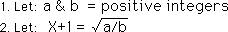Squaring both sides.Expanding, subtracting and factoring.Dividing by X+2 and simplifying.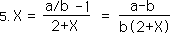Equation 2 in another form.Substituting for X on the right side of equation continuously we get the infinite continued fraction, which yields our square root.#### The F Series for the square root of a positive rational number

In the same fashion that we did for the √2, we generate the Infinite Continued Fraction Series, the F Series, by truncating Equation 7 after successive parts. After some specific examples we get a general expression for ƒ(N), the Nth member of our F Series.Notice that this is a first order iterative expression, where N represents the number of iterations.

#### The relation between the N& D Series

We break ƒ(N-1) into a fraction, i.e. a Numerator Series, n(N-1), and a Denominator Series, d(N-1), as before. We then simplify our equation.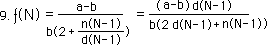We see that n(N) can be written in terms of d(N-1).#### The D Series

In equation 9, We substitute a d(N-2) for the n(N-1) as per the above equation.Now d(N) can be written and generated by the two previous members of the denominator series.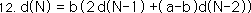ƒ(N) can now be written solely in terms of the denominator series.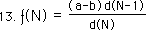#### The limit of our F Series

Remembering Equation 7, we see that the limit of our F Series as N approaches infinity is the square root of our rational Root Number - 1.Another way of writing the same thing, with a notational simplification.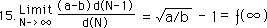#### Generating the positive denominator series

Looking back at the equations in step 8, we are able to determine the first few elements of the denominator series. Then using equation 12, we can generate the rest of the elements.Finally we are able to write the elements of the positive denominator series. A function of the ratio of the consecutive elements approaches the Limit, which is the Square Root minus one, √a/b -1.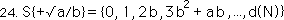#### The inverse F Series, F’

Now let us generate the inverse F Series. Solving Equation 12 for d(N-2).Replacing N-2 with N, we get an expression for N in terms of the two subsequent members of the series.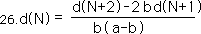We substitute this expression for d(N-1) in Equation 13, shown below.Simplifying, many terms drop out, leaving us with this expression.We let the first part be the inverse function.Substituting the inverse function into equation 28, we get this relation. It is the same relation, we derived previously for the √2 and the √a. Now however ƒ(N) and ƒ'(N) have been defined in a more general way that includes the specific cases.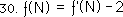Using the notation of equation 15, we get the following relation.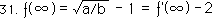Adding one to all parts of the equation we get the following symmetrical relation.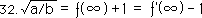Finally we see that as the inverse function approaches infinity that its value approaches the square root of the rational number + 1.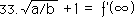### B. Square Root Theorems for √a/b

Let us now restate our basic iterative theorems in a more general fashion that will include the square root of any positive rational number rather than just the integers.

#### First Theorem: The F Series Theorem for the square root of any positive rational number, a/b.

Let us extend our basic iterative theorems by restating them in terms of rational square roots. Let us begin with the First Theorem, i.e. the F Series Theorem, for the square root of any positive rational number, a/b.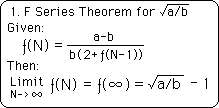Notice that this theorem does not contradict the F Series for integers. It only extends it to more cases. When we have an integer, then the denominator, b, equals one, turning it into our initial F Series expression, where the coefficient of the denominator was merely one.

#### Second Theorem: The N Series Theorem for the square root of any positive rational number, a/b.

Again we restate our Second Theorem, the Numerator Theorem, which is important because of its ability to neutralize the N Series as a factor in determining the F Series.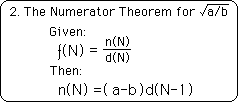Notice that once again the original formulation of the Numerator Series is not contradicted, but merely extended.

#### Third Theorem: The D Series for the square root of any positive rational number, a/b.

The Third Theorem, the Denominator Series Theorem, as before, first tells how to generate the D Series. Then it connects the D Series with the F Series and its Limit.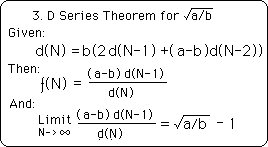We have another extension of previous results, not a contradiction. These three basic theorems of our Iterative Root Family have now been extended to apply to the square root of any positive rational number.

#### Fourth Theorem: the Inverse F Series Theorem for positive rational numbers, a/b

There are also some theorems associated with the inverse F Series, which need to be extended also. The Fourth Theorem is the Inverse F Series Theorem. It defines the inverse series and states what its limit is.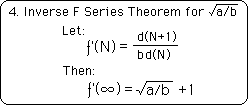These results come from equations 29 and 33.

#### Inverse F Series means reverse D Series ratio

Note that it is called the Inverse F Series because it is generated by a function of the reverse ratio of the D Series, not because the product of the F Series and the Inverse F Series equals one. In fact their product does not equal one, as we shall soon see.

#### Difference Theorems, A & B, for the square root of any positive rational numbers, a/b

We also generalize the Difference Theorems, A & B, for the square roots of any positive rational numbers, a/b. These relate the finite member of the F and F’ Series in a simple difference, which equals 2. This result comes from equation 30. It also relates the limit of the F and F’ Series in the same simple difference which also equals two. This comes from equation 32.#### Experimental verification stronger again

Again these theorems have been tested experimentally via the computer. While the past tests were for a random smattering of integers, this series of tests took a random smattering of rational numbers. Again each of these tested out surprisingly accurately. The accidental generation of 16 places of accuracy for the square root of a random assortment of rational numbers including 3/2, 13/5, or 27/7 is impossible, practically speaking. Therefore the experimental verification of our Iterative Root Theorems is greatly strengthened by adding the class of positive rational numbers to the family.

### C. Summarizing the discoveries

#### The Negative Denominator Series only applies to √2

We discovered a Negative Denominator Series For the √2. It generates integers that grow towards positive and negative infinity. The ratio of consecutive members of this series approximates plus or minus the square root of 2 plus or minus one, depending upon whether one goes to positive or negative infinity, with the ratio one way or the other. While this symmetrical number square based upon the negative D Series works well with the square root of 2, it does not apply to any other number. The relative symmetry of the D Series for √2, is immediately shattered by the (a-1) term that is introduced for integers. Thus the negative D Series in all it symmetrical beauty only exists for the D Series for √2, not for any others. Lets hear it for the special qualities of individuality, which do not extend to the general, but are allowed by it.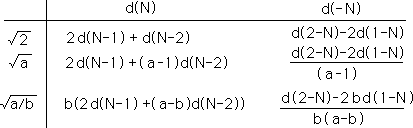A table of the D Series for the √2, √a, and the √a/b with their corresponding negative D Series is shown above. Note the increasing complexity of the coefficients of the D Series. Note that they are all based upon two layers of feedback and that the generalizations are extensions, not contradictions. Further the positive D Series always generate integers. On the other hand, the negative D Series, after the √2 turn fractional. This is because of the b(a-b) factor in the denominator. These negative series do not generate any limit. This is an example of the individual differing from the general.

#### The difference between reciprocal functions is always 2

Below is a chart which identifies the F and F’ Series for √2, √a, and √a/b. Unusually the difference in the inverse functions is always 2. The functions are based upon the ratio between consecutive elements in the Denominator series. The inverse functions are based upon the same ratio in reverse order. It is easy to see that the general rational function reduces to the integer function when b=1. Also the integer function reduces to the specific function for the √2 when a = 2.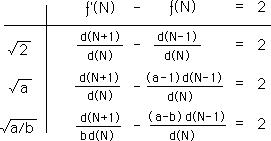#### The meaning of the functions

The limit of these functions as N approaches infinity plus or minus one are the square roots in mention. This ties these series to something real and gives them meaning. The functions were derived from the square roots and determine the square roots.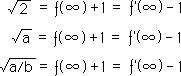#### Interconnected concepts

Our theorems reveal a high degree of interconnectedness. The definitions of the F Series are derived from the infinite continued fractions, which determine the square root of any rational number. While the Denominator Series is derived from the Infinite Continued Fraction Series, they can also be used to determine it. Simplicity in the midst of complexity.